International
Tables for
Crystallography
Volume A
Space-group symmetry
Edited by M. I. Aroyo

International Tables for Crystallography (2016). Vol. A, ch. 1.3, p. 31

## Section 1.3.3.3. Symmorphic and non-symmorphic space groups

B. Souvigniera*

aRadboud University Nijmegen, Faculty of Science, Mathematics and Computing Science, Institute for Mathematics, Astrophysics and Particle Physics, Postbus 9010, 6500 GL Nijmegen, The Netherlands
Correspondence e-mail: souvi@math.ru.nl

#### 1.3.3.3. Symmorphic and non-symmorphic space groups

| top | pdf |

If a coset with respect to the translation subgroup contains an operation of the formwitha vector in the translation lattice, it is clear that the same coset also contains the operation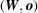with trivial translation part. On the other hand, if a coset does not contain an operation of the form, this may be caused by an inappropriate choice of origin. For example, the operation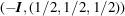is turned into the inversion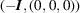by moving the origin to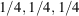(cf. Section 1.5.1.1for a detailed treatment of origin-shift transformations).

Depending on the actual space group, it may or may not be possible to choose the origin such that every coset with respect tocontains an operation of the form.

#### Definition

Letbe a space group with translation subgroup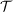. If it is possible to choose the coordinate system such that every coset ofwith respect tocontains an operationwith trivial translation part,is called a symmorphic space group, otherwiseis called a non-symmorphic space group.

One sees that the operations with trivial translation part form a subgroup ofwhich is isomorphic to a subgroup of the point group. This subgroup is the group of operations inthat fix the origin and is called the site-symmetry group of the origin (site-symmetry groups are discussed in detail in Section 1.4.4). It is the distinctive property of symmorphic space groups that they contain a subgroup which is isomorphic to the full point group. This may in fact be seen as an alternative definition for symmorphic space groups.

Proposition. A space groupwith point groupis symmorphic if and only if it contains a subgroup isomorphic to. For a non-symmorphic space group, every finite subgroup ofis isomorphic to a proper subgroup of the point group.

Note that every finite subgroup of a space group is a subgroup of the site-symmetry group for some point, because finite groups cannot contain translations. Therefore, a symmorphic space group is characterized by the fact that it contains a site-symmetry group isomorphic to its point group, whereas in non-symmorphic space groups all site-symmetry groups have orders strictly smaller than the order of the point group.

Symmorphic space groups can easily be constructed by choosing a lattice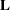and a point groupwhich acts on. Then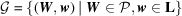is a space group in which the coset representatives can be chosen as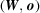.

Non-symmorphic space groups can also be constructed from a latticeand a point group. What is required is a system of coset representatives with respect toand these are obtained by choosing for each operation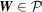a translation part. Owing to the translations, it is sufficient to consider vectorswith components between 0 and 1. However, the translation parts cannot be chosen arbitrarily, because for a point-group operation of order k, the operation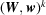has to be a translation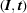with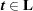. Working this out, this imposes the restriction that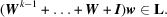Once translation partsare found that fulfil all these restrictions, one finally has to check whether the space group obtained this way is (by accident) symmorphic, but written with respect to an inappropriate origin. A change of origin by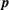is realized by conjugating the matrix–column pairby the translation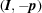(cf. Section 1.5.1on transformations of the coordinate system) which gives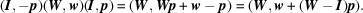Thus, the space group just constructed is symmorphic if there is a vectorsuch that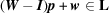for each of the coset representatives.

The above considerations also show how every space group can be assigned to a symmorphic space group in a canonical way, namely by setting the translation parts of coset representatives with respect toto. This has the effect that screw rotations are turned into rotations and glide reflections into reflections. The Hermann–Mauguin symbol (see Section 1.4.1for a detailed discussion of Hermann–Mauguin symbols) of the symmorphic space group to which an arbitrary space group is assigned is simply obtained by replacing any screw rotation symbol Nm by the corresponding rotation symbol N and every glide reflection symbol a, b, c, d, e, n by the symbol m for a reflection. A space group is found to be symmorphic if no such replacement is required, i.e. if the Hermann–Mauguin symbol only contains the symbols 1, 2, 3, 4, 6 for rotations,,,,for rotoinversions and m for reflections.

#### Example

The space groups with Hermann-Mauguin symbols P4mm, P4bm, P42cm, P42nm, P4cc, P4nc, P42mc, P42bc are all assigned to the symmorphic space group with Hermann–Mauguin symbol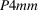.